# 2. 有限混合模型的EM算法

EM算法迭代最大化，而不是观察到的对数似然Lx(θ)，算式为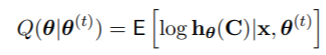1. E步：计算Q(θ|θ(t))
2. M步骤：设定θ(t+1)=argmaxθ∈Φ Q(θ|θ(t))

KMEANS均值聚类和层次聚类：R语言分析生活幸福质量系数可视化实例

Z是离散的，它们的分布是通过贝叶斯定理给出的。M步骤本身可以分成两部分，与λ有关的最大化，它不依赖于F，与φ有关的最大化，它必须为每个模型专门处理（例如，参数化、半参数化或非参数化）。因此，模型的EM算法有以下共同特点。
11. E步。计算成分包含的 “后验 “概率（以数据和θ（t）为条件）。

2.  λ的M步骤。设

# 基于模型的聚类和R语言中的高斯混合模型

## 2.3. 一个EM算法的例子

``mixEM(waiting, lambda = .5)``

### 随时关注您喜欢的主题

``````R> plot(wait1, density = TRUE, cex.axis = 1.4, cex.lab = 1.4, cex.main = 1.8,
+ main2 = "Time between Old Faithful eruptions", xlab2 = "Minutes")``````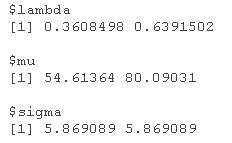``summary(wait1)``

# 3. Cutpoint methods切割点方法

``````R> cutpts <- 10.5*(-6:6)
R> mult(data, cuts = cutpts)``````

# 一旦创建了多指标数据，我们可以应用EM算法估计多指标参数。最后，计算并绘制出方程的估计分布函数。

``````R> plot(data, posterior, lwd = 2,
+ main = "三分量解")``````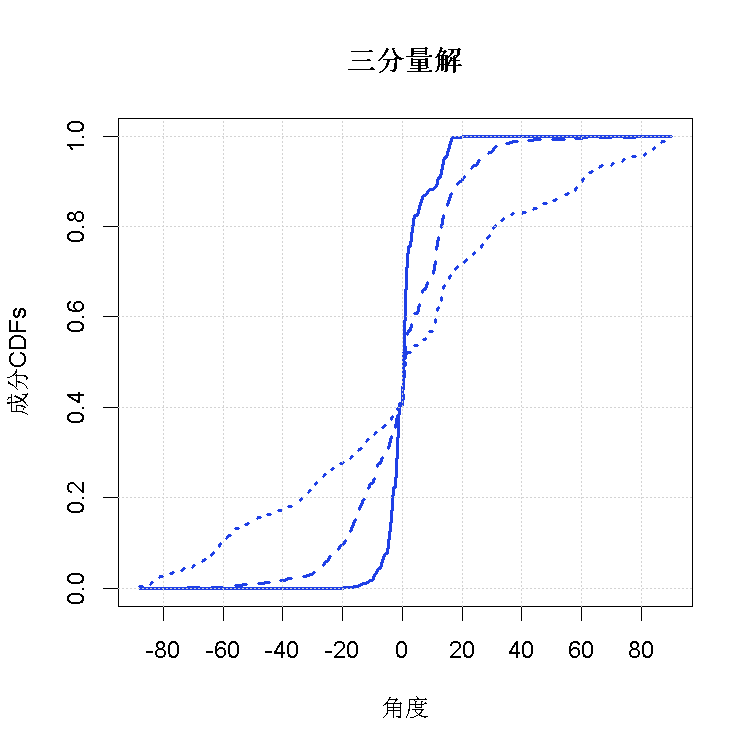图3(a)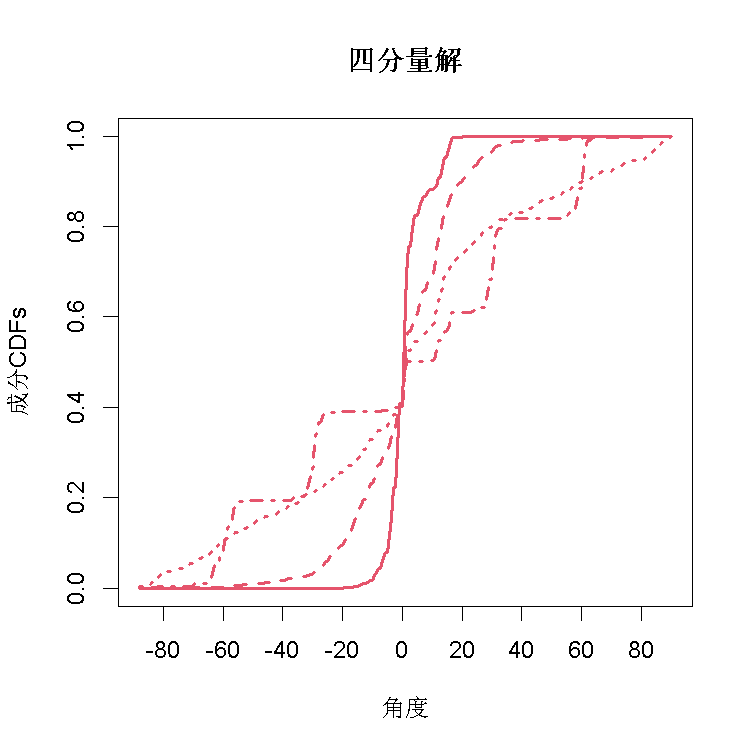图3(b)

# 单变量对称、位置偏移的半参数例子

``````R> plot(wait1, which = 2 )
R> wait2 <-EM(waiting)
R> plot(wait2, lty = 2)``````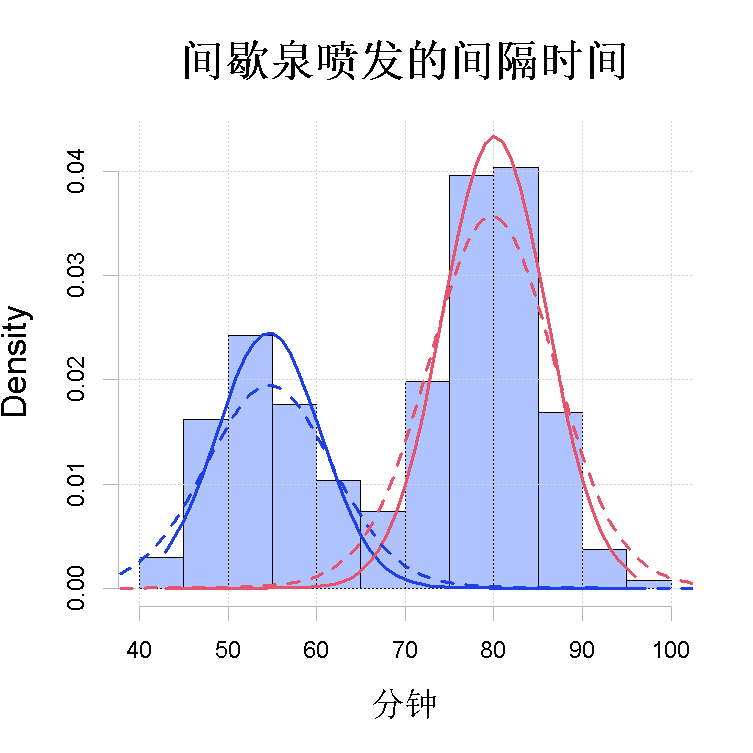图4(a)

``R> bw.nrd0(wait)``

``````> wait2a <- EM(wait, bw = 1)
> plot(wait2a
> plot(wait2b``````

Kaizong Ye拓端研究室（TRL）的研究员。

​非常感谢您阅读本文，如需帮助请联系我们！QQ在线咨询

15121130882

0571-63341498

## 关注有关新文章的微信公众号

This will close in 0 seconds